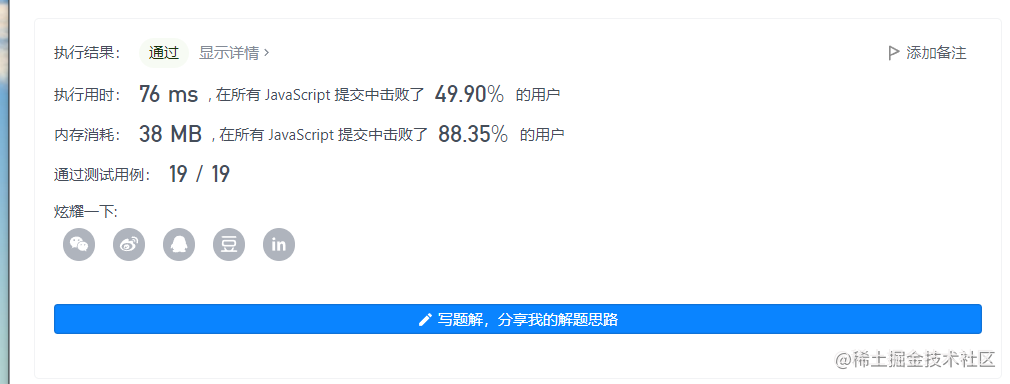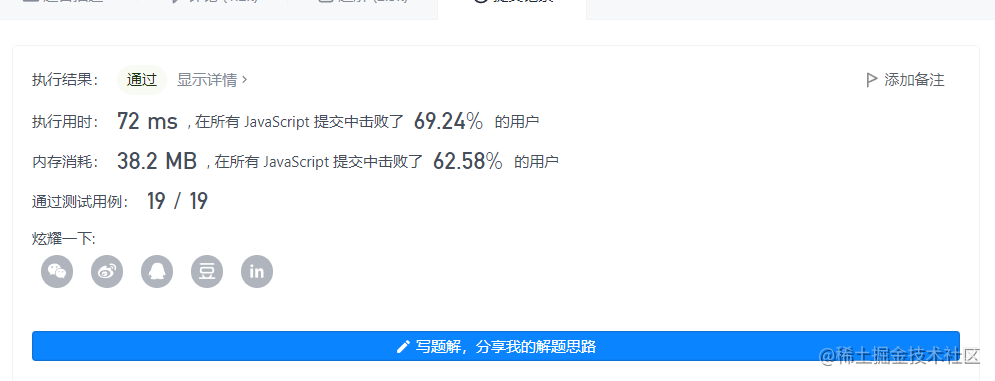2022-11-1700

1. 题目
2. 前言
3.二分查找
4. 双指针

# 1. 题目

``````输入：numbers = [2,7,11,15], target = 9

``````

``````输入：numbers = [2,3,4], target = 6

``````

``````输入：numbers = [-1,0], target = -1

``````

• 2 <= numbers.length <= 3 * 104
• -1000 <= numbers[i] <= 1000
• numbers 按 非递减顺序 排列
• -1000 <= target <= 1000
• 仅存在一个有效答案
• 通过次数345,963提交次数58

# 3.二分查找

```javascript```/**
* @param {number[]} numbers
* @param {number} target
* @return {number[]}
*/
var twoSum = function(numbers, target) {
const len = numbers.length
for(let i = 0; i < len - 1; i++) {
let left = i + 1
let right = len - 1
const needNum = target - numbers[i]
while(left <= right) {
const mid = (left + right) >> 1
if(numbers[mid] === needNum) {
return [i + 1, mid + 1]
}else if(numbers[mid] < needNum) {
left = mid + 1
} else if(numbers[mid] > needNum) {
right = mid - 1
}
}
}
};
``````

• 时间复杂度：O(nlogn)
• 空间复杂度：O(1)# 4. 双指针

```JAVASCRIPT```/**
* @param {number[]} numbers
* @param {number} target
* @return {number[]}
*/
var twoSum = function(numbers, target) {
let left = 0,right = numbers.length - 1
while(left < right) {
const sum = numbers[left] + numbers[right]
if(sum > target) {
right--
} else if(sum < target) {
left++
} else {
return [left + 1, right + 1]
}
}
};
``````

• 时间复杂度：O(n)，其中 nn 是数组的长度。两个指针移动的总次数最多为 n 次。
• 空间复杂度：O(1)。